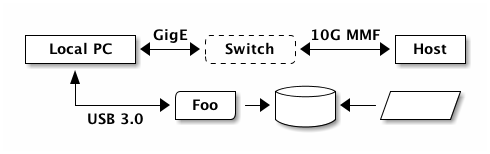This is how I remember all the stuff I can do with Jekyll/Markdown/Kramdown/ditaa/MathJax/stuff.

Test post pls ignore. Test post pls ignore. Test post pls ignore.

Crossed out thing

• List
• Of
• Things (with splats)

• List
• Of
• Other Things (with hyphens)
• Nested
• Things
• Double
• Nested
• Even
• More
1. Numbered
2. List
3. With Numbers
1. Nested
2. Numbers
4. Wrong Number
• Not
• Numbered

jekyll-responsive-imagedef what?
42
end

Here comes some code

This one is separate.

Default aligned Left aligned Center aligned Right aligned
First body part Second cell Third cell fourth cell
Second line foo strong baz
Third line quux baz bar
Second body
2 line
Footer row

A nice blockquote With multiple lines

This is a text with a footnote1. This is more text with a footnote2.

This is an acronym example: NASA

PFD is an acronym also.

Kramdown Quick Reference

ditaa test:MathJax block:

MathJax inline: $$\alpha = \left(1 + \frac{\text{FRAC}}{\text{MOD}} \right) + \int_{-1}^{\infty} \beta \ dx$$

1. And here is the definition.

2. Here is where stuff about footnote 2 goes.